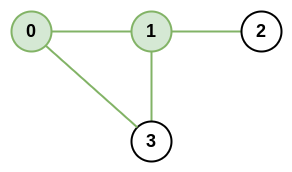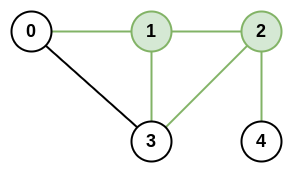1615. Maximal Network Rank
Medium
1.1K
190

There is an infrastructure of `n` cities with some number of `roads` connecting these cities. Each `roads[i] = [ai, bi]` indicates that there is a bidirectional road between cities `ai` and `bi`.

The network rank of two different cities is defined as the total number of directly connected roads to either city. If a road is directly connected to both cities, it is only counted once.

The maximal network rank of the infrastructure is the maximum network rank of all pairs of different cities.

Given the integer `n` and the array `roads`, return the maximal network rank of the entire infrastructure.

Example 1:```Input: n = 4, roads = [[0,1],[0,3],[1,2],[1,3]]
Output: 4
Explanation: The network rank of cities 0 and 1 is 4 as there are 4 roads that are connected to either 0 or 1. The road between 0 and 1 is only counted once.
```

Example 2:```Input: n = 5, roads = [[0,1],[0,3],[1,2],[1,3],[2,3],[2,4]]
Output: 5
Explanation: There are 5 roads that are connected to cities 1 or 2.
```

Example 3:

```Input: n = 8, roads = [[0,1],[1,2],[2,3],[2,4],[5,6],[5,7]]
Output: 5
Explanation: The network rank of 2 and 5 is 5. Notice that all the cities do not have to be connected.
```

Constraints:

• `2 <= n <= 100`
• `0 <= roads.length <= n * (n - 1) / 2`
• `roads[i].length == 2`
• `0 <= ai, bi <= n-1`
• `ai != bi`
• Each pair of cities has at most one road connecting them.
Accepted
60.8K
Submissions
103.8K
Acceptance Rate
58.5%

Seen this question in a real interview before?
1/4
Yes
No

Discussion (0)

Related Topics